# Show all your work, thanks! 18. A sample of helium gas (mass of 2.658x10-3kg), is expanded...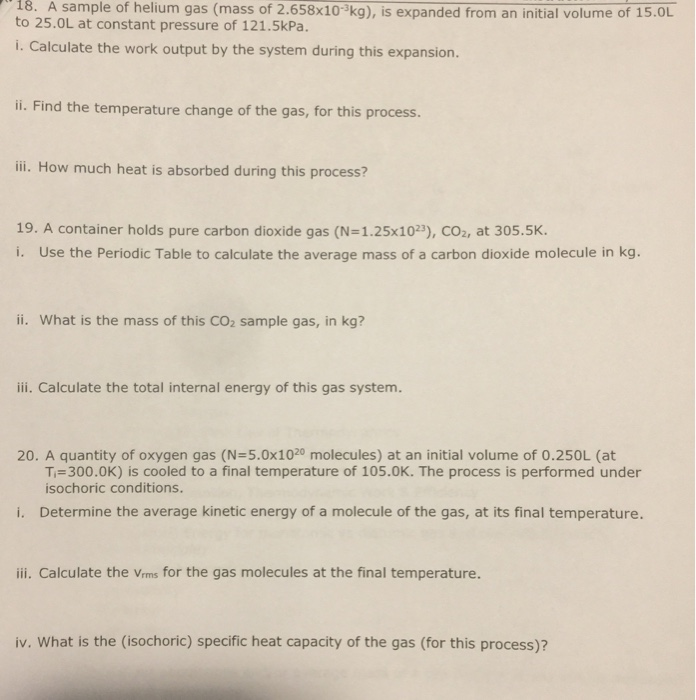18. A sample of helium gas (mass of 2.658x10-3kg), is expanded from an initial volume of 15.OL to 25.OL at constant pressure of 121.5kPa. i. Calculate the work output by the system during this expansion. i. Find the temperature change of the gas, for this process. ili. How much heat is absorbed during this process? 19. A container holds pure carbon dioxide gas (N=1.25x10», СОг, at 305.5K. i. Use the Periodic Table to calculate the average mass of a carbon dioxide molecule in kg. ii. What is the mass of this CO2 sample gas, in kg? iii. Calculate the total internal energy of this gas system 20. A quantity of oxygen gas (N=5.0x1020 molecules) at an initial volume of 0.250L (at T,=300.0K) is cooled to a final temperature of 105.0K. The process is performed under isochoric conditions Determine the average kinetic energy of a molecule of the gas, at its final temperature. i. ii. Calculate the Vrms for the gas molecules at the final temperature. iv. What is the (isochoric) specific heat capacity of the gas (for this process)?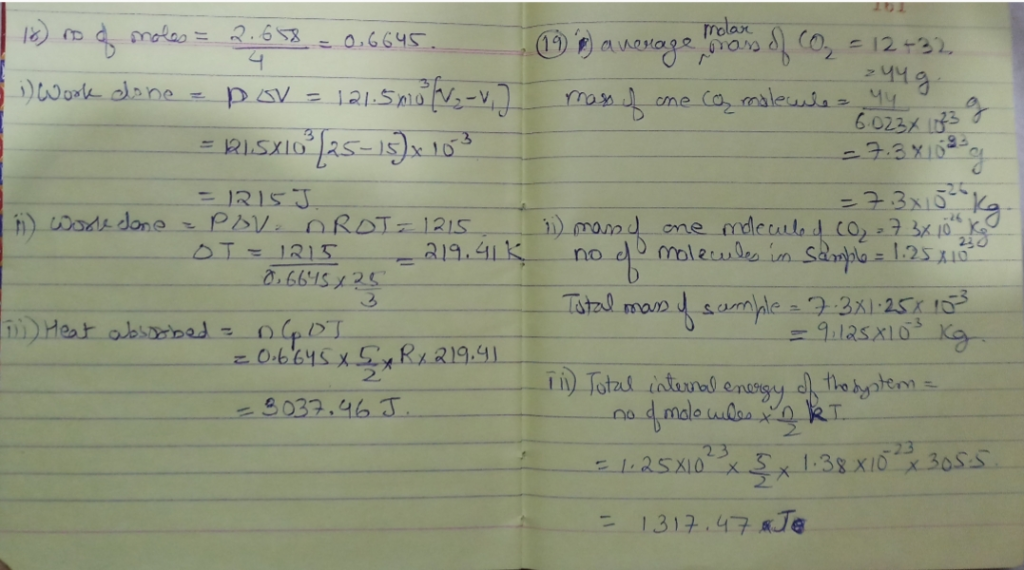#### Earn Coin

Coins can be redeemed for fabulous gifts.

Similar Homework Help Questions
• ### An isolated gas (nitrogen, N2), at initial temperature of 22.7 degrees Celsius, is expanded from as...

An isolated gas (nitrogen, N2), at initial temperature of 22.7 degrees Celsius, is expanded from as initial volume of 0.750L to 1.450L at constant pressure, P=132kPa. During this process the gas absorbs 318.5J of heat. i. Calculate the work output by the system during this expansion ii. Estimate the specific heat capacity of the gas for this process. Express your answer in J/kg*K.

• ### Please answer question 6. thanks 1. A 7 kg lead sphere that has 35 °C temperature...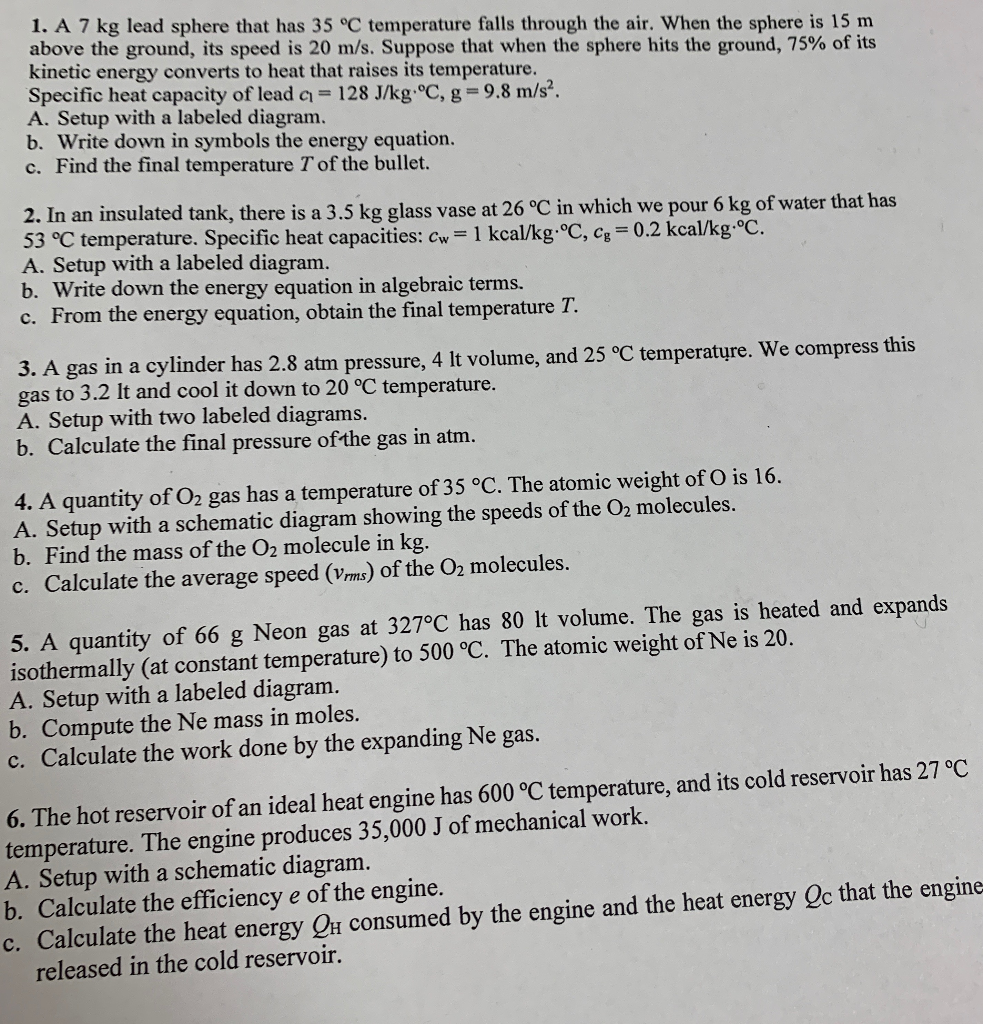Please answer question 6. thanks 1. A 7 kg lead sphere that has 35 °C temperature falls through the air. When the sphere is 15 m above the ground, its speed is 20 m/s. Suppose that when the sphere hits the ground, 75% of its kinetic energy converts to heat that raises its temperature. Specific heat capacity of lead c = 128 J/kg.°C, g=9.8 m/s. A. Setup with a labeled diagram. b. Write down in symbols the energy equation. c....

• ### Carbon dioxide gas is contained in a rigid vessel (internal volume-200 liters, mass-10 kg. Cp= 0.47...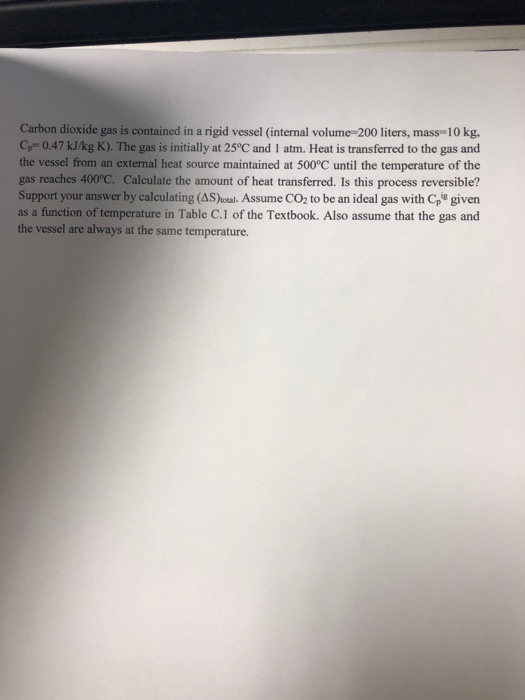Carbon dioxide gas is contained in a rigid vessel (internal volume-200 liters, mass-10 kg. Cp= 0.47 kJ/kg K). The gas is initially at 25°C and 1 atm. Heat is transferred to the gas and the vessel from an external heat source maintained at 500°C until the temperature of the gas reaches 400°C. Calculate the amount of heat transferred. Is this process reversible? Support your answer by calculating (AS)iotal. Assume CO2 to be an ideal gas with Cp given as a...

• ### Please answer all parts of the Question: a,b,c,d Kinetic Theory of Gas: Explanation of Pressure and...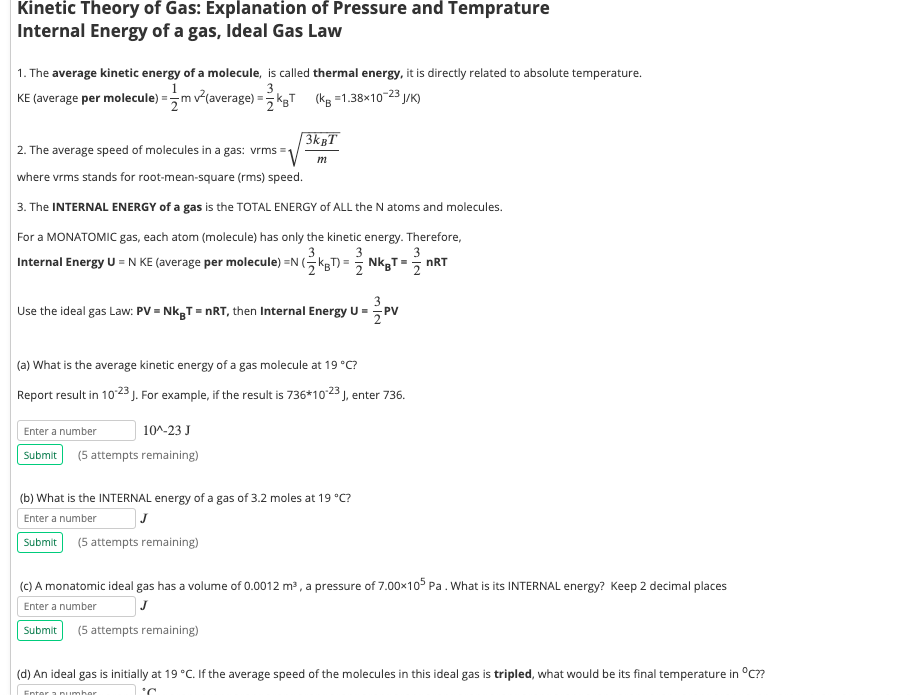Please answer all parts of the Question: a,b,c,d Kinetic Theory of Gas: Explanation of Pressure and Temprature Internal Energy of a gas, Ideal Gas Law 1. The average kinetic energy of a molecule, is called thermal energy, it is directly related to absolute temperature. KE (average per molecule) = 5m +(average) = 1 kg(kp = 1.38x10-23 /K) KT 2. The average speed of molecules in a gas: vrms=1 where vrms stands for root-mean-square (rms) speed. 3. The INTERNAL ENERGY of...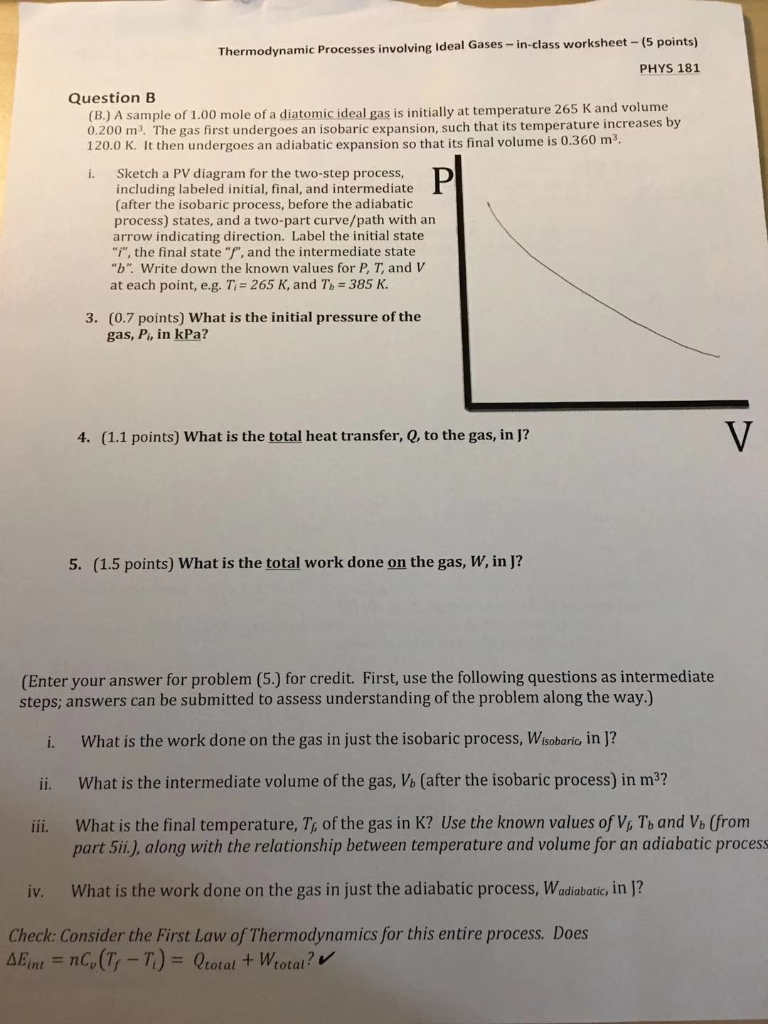Please help me about Physics, Thanks. A sample of 1.00 mole of a diatomic ideal gas is intially at temperature 265K........... Thermodynamic Processes involving Ideal Gases-in-class worksheet-(5 points) PHYS 181 Question B (B.) A sample of 1.00 mole of a diatomic ideal gas is initially at temperature 265 K and volume 0.200 m. The gas first undergoes an isobaric expansion, such that its temperature increases by 120.0 K. It then undergoes an adiabatic expansion so that its final volume is...

• ### Please help and show work. Thanks! (3). A sample of 1.00 mol ideal gas molecules with...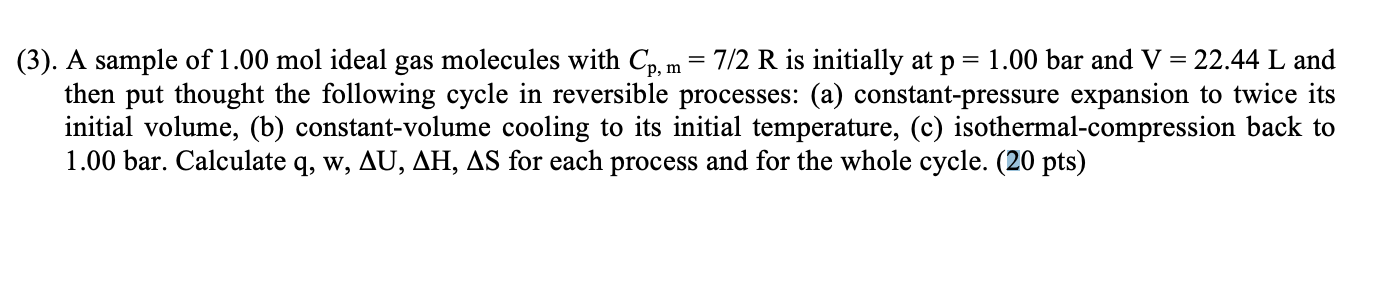Please help and show work. Thanks! (3). A sample of 1.00 mol ideal gas molecules with Cp, m = 7/2 R is initially at p = 1.00 bar and V = 22.44 L and then put thought the following cycle in reversible processes: (a) constant-pressure expansion to twice its initial volume, (b) constant-volume cooling to its initial temperature, (c) isothermal-compression back to 1.00 bar. Calculate q, w, AU, AH, AS for each process and for the whole cycle. (20 pts)

• ### PLEASE SHOW ALL YOUR WORK CLEARLY, AND EXPLAIN YOUR PROCESS. DATA: Initial mass of cup with...

PLEASE SHOW ALL YOUR WORK CLEARLY, AND EXPLAIN YOUR PROCESS. DATA: Initial mass of cup with water = 99.69 g Final mass of cup with water = 150.89 g Initial temperature of water = 41 °C Final temperature of water = 4.4 °C Analysis a) Determine the mass of the ice that has melted. b) Determine the variation of the water temperature (ΔT). c) Calculate the energy (in J) released by 100 g of water when it cooled by ΔT....

• ### 4) An ideal-gas mixture of helium and nitrogen with a nitrogen mass fraction of 35 percent...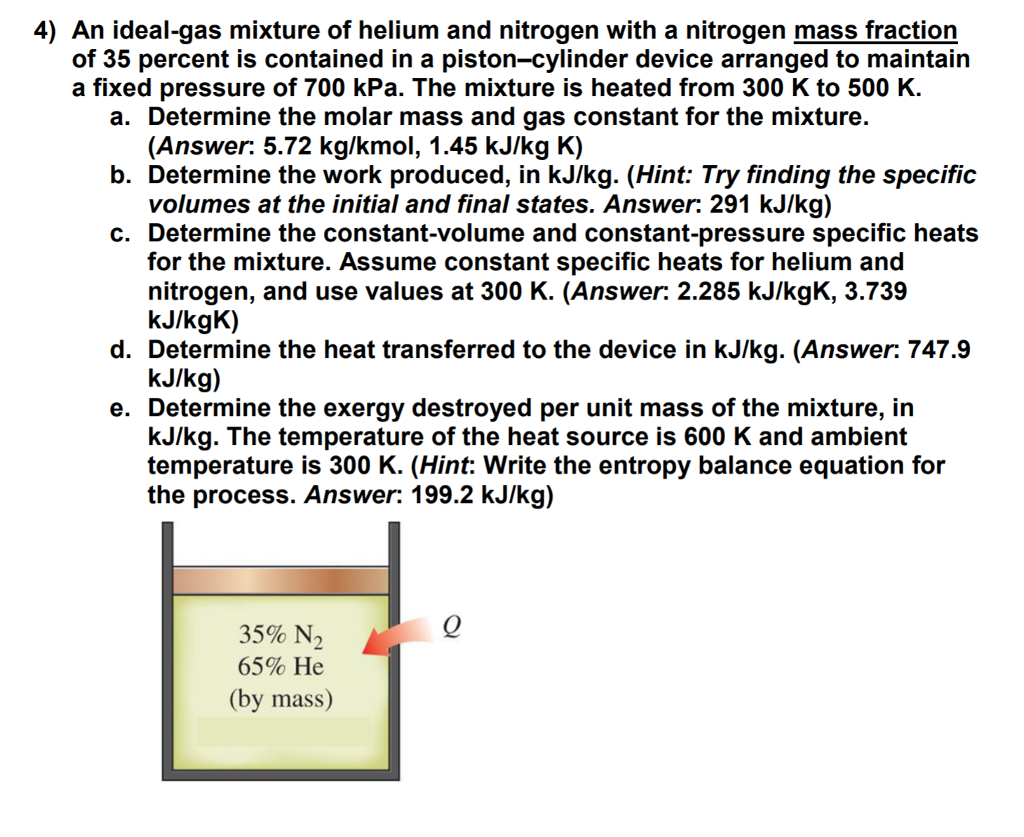4) An ideal-gas mixture of helium and nitrogen with a nitrogen mass fraction of 35 percent is contained in a piston-cylinder device arranged to maintain a fixed pressure of 700 kPa. The mixture is heated from 300 K to 500 K a. Determine the molar mass and gas constant for the mixture. b. Determine the work produced, in kJ/kg. (Hint: Try finding the specific c. Determine the constant-volume and constant-pressure specific heats (Answer: 5.72 kg/kmol, 1.45 kJ/kg K) volumes at...

• ### PLEASE SOLVE ALL QUESTIONS ( EMPHASIZE ON D + E ) PLEASE ( CLEAR HANDWRITING) Question...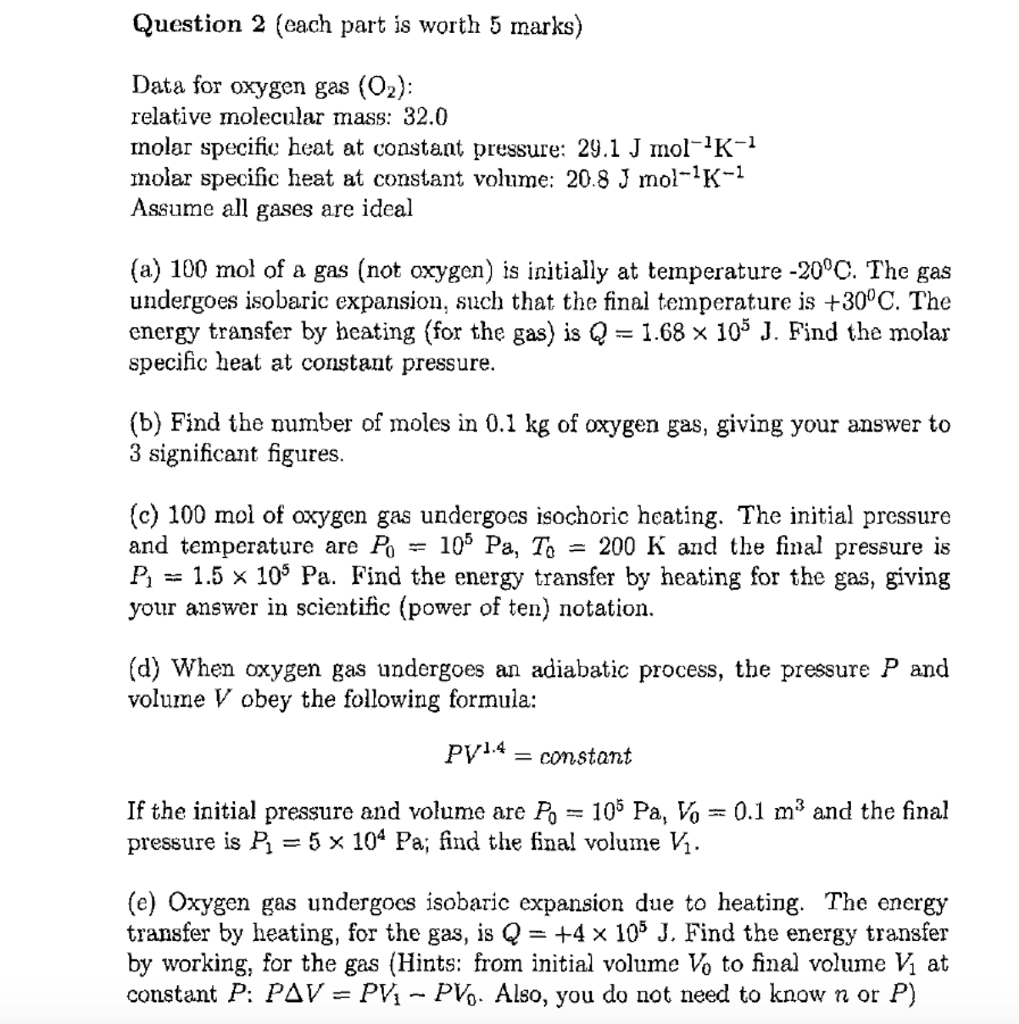PLEASE SOLVE ALL QUESTIONS ( EMPHASIZE ON D + E ) PLEASE ( CLEAR HANDWRITING) Question 2 (each part is worth 5 marks) Data for oxygen gas (02): relative molecular mass: 32.0 molar specific heat at constant pressure: 29.1 J nol-1K-1 molar specific heat at constant volume: 20.8 J mol-1K-1 Assume all gases are ideal (a) 100 mol of a gas (not oxygen) is initially at temperature -20°C. The gas undergoes isobaric expansion, such that the final temperature is +300C....

• ### A sample of Anurance Cound is van O STATES OF MATTER Interconverting molar mass and density...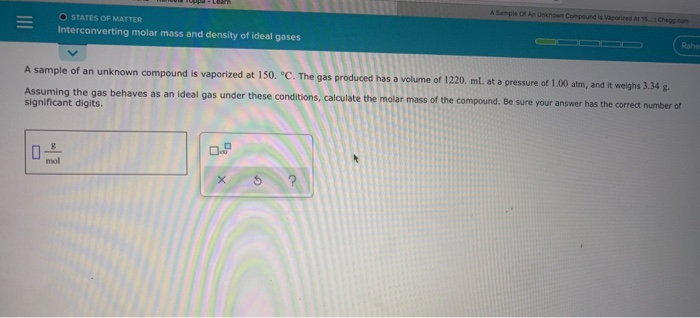A sample of Anurance Cound is van O STATES OF MATTER Interconverting molar mass and density of ideal gases Raho A sample of an unknown compound is vaporized at 150. °C. The gas produced has a volume of 1220 mL at a pressure of 1.00 atm, and it weighs 3.34 g. Assuming the gas behaves as an ideal gas under these conditions, calculate the molar mass of the compound. Be sure your answer has the correct number of significant digits....

Free Homework App